Tuesday, June 22, 2021
Home > IMO > Pre RMO Previous Year 2013 Question Paper With Solutions

# Pre RMO Previous Year 2013 Question Paper With Solutions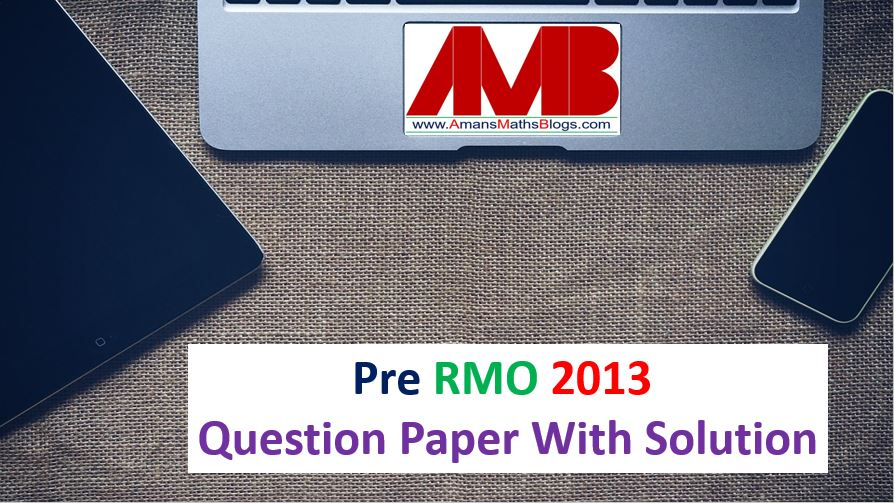# Pre RMO Previous Year 2013 Question Paper With Solutions

In this post, you will get Pre RMO Previous Year 2013 Question Paper With Solutions.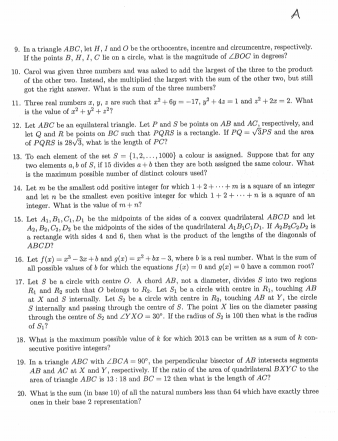Pre RMO Previous Year 2013 Question Paper Question No 1:

What is the smallest positive integer k such that k(33 + 43 + 53) = an for some positive integers a and n, with n > 1?

Pre RMO Previous Year 2013 Question Paper with Solutions of Question No 1:Pre RMO Previous Year 2013 Question Paper Question No 2:

Let Sn =. What is the value of.

Pre RMO Previous Year 2013 Question Paper Solution of Question No 2: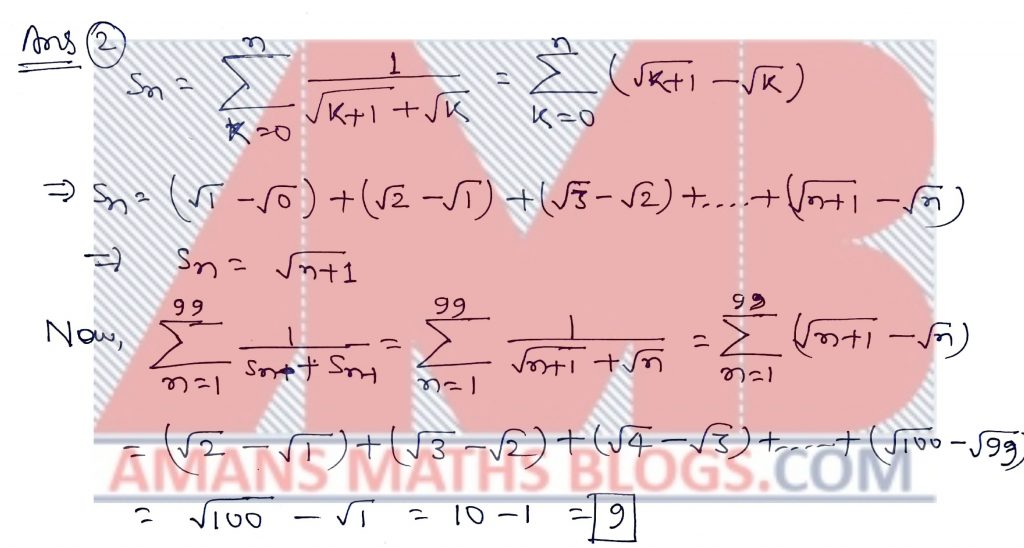Pre RMO Previous Year 2013 Question Paper Question No 3:

It is given that the equation x2 + ax + 20 = 0 has integer roots. What is the sum of all possible values of a?

Pre RMO Previous Year 2013 Question Paper Solution of Question No 3: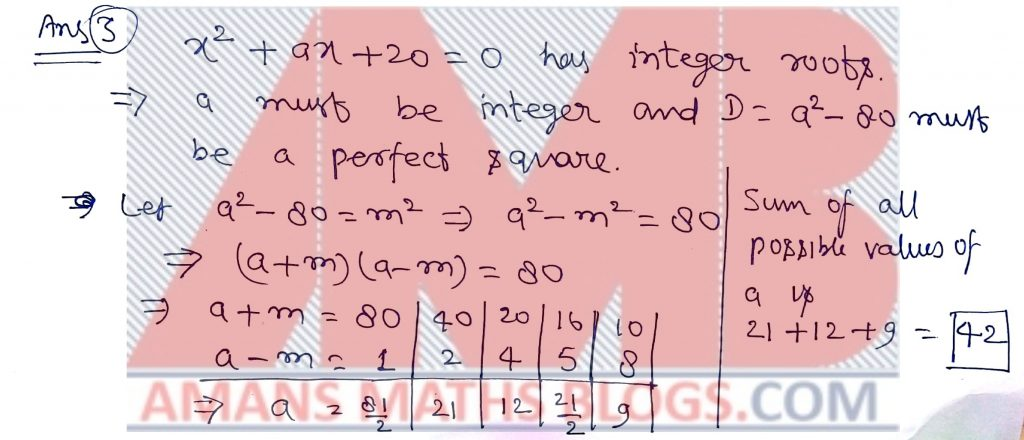Pre RMO Previous Year 2013 Question Paper Question No 4:

Three points X, Y, Z are on a straight line such that XY = 10 and XY = 3. What is the product of all possible values of YZ?

Pre RMO Previous Year 2013 Question Paper Solution of Question No 4:Pre RMO Previous Year 2013 Question Paper Question No 5:

There are n – 1 red balls, n green balls and n + 1 blue balls in a bag. The numbers of ways of choosing two balls from the bag that have different colours is 299. What is the value of n?

Pre RMO Previous Year 2013 Question Paper Solution of Question No 5:Pre RMO Previous Year 2013 Question Paper Question No 6:

Let S(M) denote the sum of the digits of a positive integer M written in base 10. Let N be the smallest positive integer such that S(N) = 2013. What is the value of S(5N + 2013)?

Pre RMO Previous Year 2013 Question Paper Solution of Question No 6: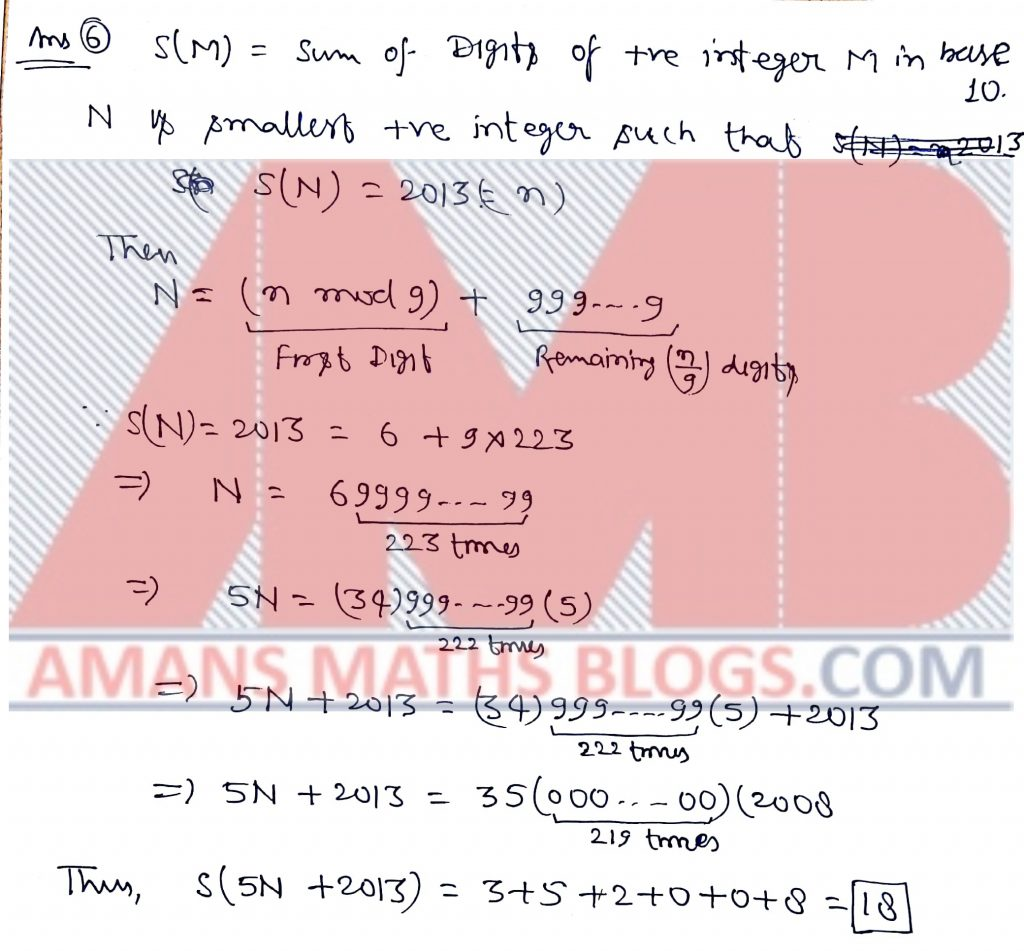Pre RMO Previous Year 2013 Question Paper Question No 7:

Let Akbar and Birbal together have n marbles, Where n>0.

Akbar says to Birbal, “If I give you some marbles then you will have twice as many marbles as I will have.” Birbal says to Akbar, “If I give you some marbles then you will have thrice as many marbles as I will have.”

What is the minimum possible value of n for which the above statements are true?

Pre RMO Previous Year 2013 Question Paper Solution of Question No 7: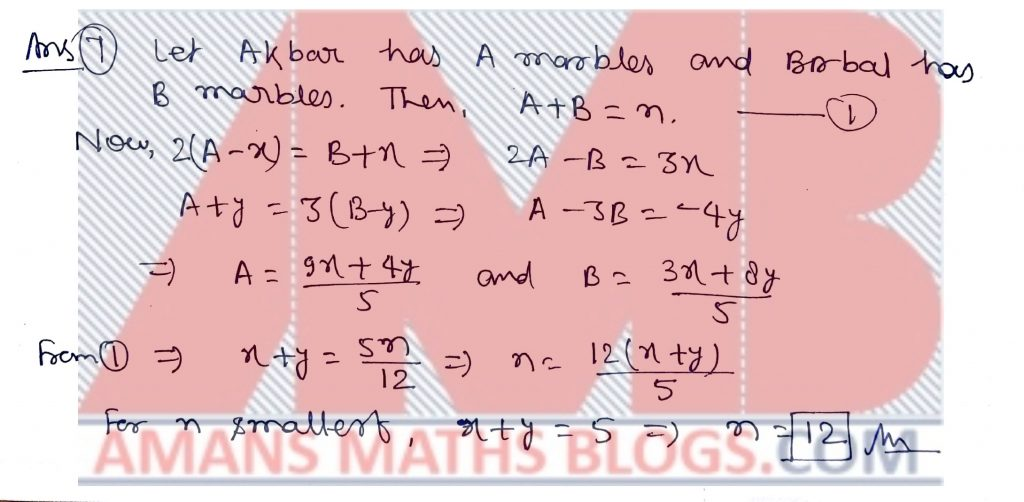Pre RMO Previous Year 2013 Question Paper Question No 8:

Let AD and BC be the parallel sides of a trapezium ABDC. Let P and Q be the midpoints of the diagonals AC and DB. If AD = 16 and BC = 20, what is the length of PQ?

Pre RMO Previous Year 2013 Question Paper Solution of Question No 8:Pre RMO Previous Year 2013 Question Paper Question No 9:

In a triangle ABC, let H, I and O be the orthocenter, incentre and circumcenter, respectively. If the points B, H, I, C lie on a circle, what is the magnitude of angle BOC in degrees?

Pre RMO Previous Year 2013 Question Paper Solution of Question No 9:Pre RMO Previous Year 2013 Question Paper Question No 10:

Carol was given three numbers and asked to add the largest of the three to the product of the other two. Instead, she multiplied the largest with the sum of the other two, but still got the right answer. What is the sum of the three numbers?

Pre RMO Previous Year 2013 Question Paper Solution of Question No 10: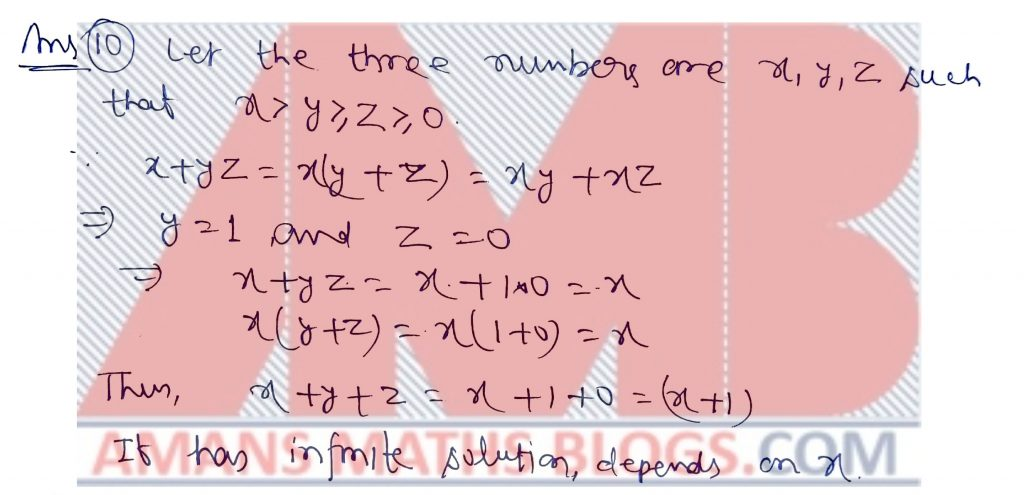Pre RMO Previous Year 2013 Question Paper Question No 11:

Three real numbers x, y, z are such that x2 + 6y = -17, y2 + 4x = 1 and z2 + 2x = 2. What is the value of x2 + y2 + z2?

Pre RMO Previous Year 2013 Question Paper Solution of Question No 11:Pre RMO Previous Year 2013 Question Paper Question No 12:

Let ABC be an equilateral triangle. Let P and S be points on AB and AC, respectively, and let Q and R be points on BC such that PQRS is a rectangle. If PQ =PS And the area of PQRS is 28, what is the length of PC?

Pre RMO Previous Year 2013 Question Paper Solution of Question No 12: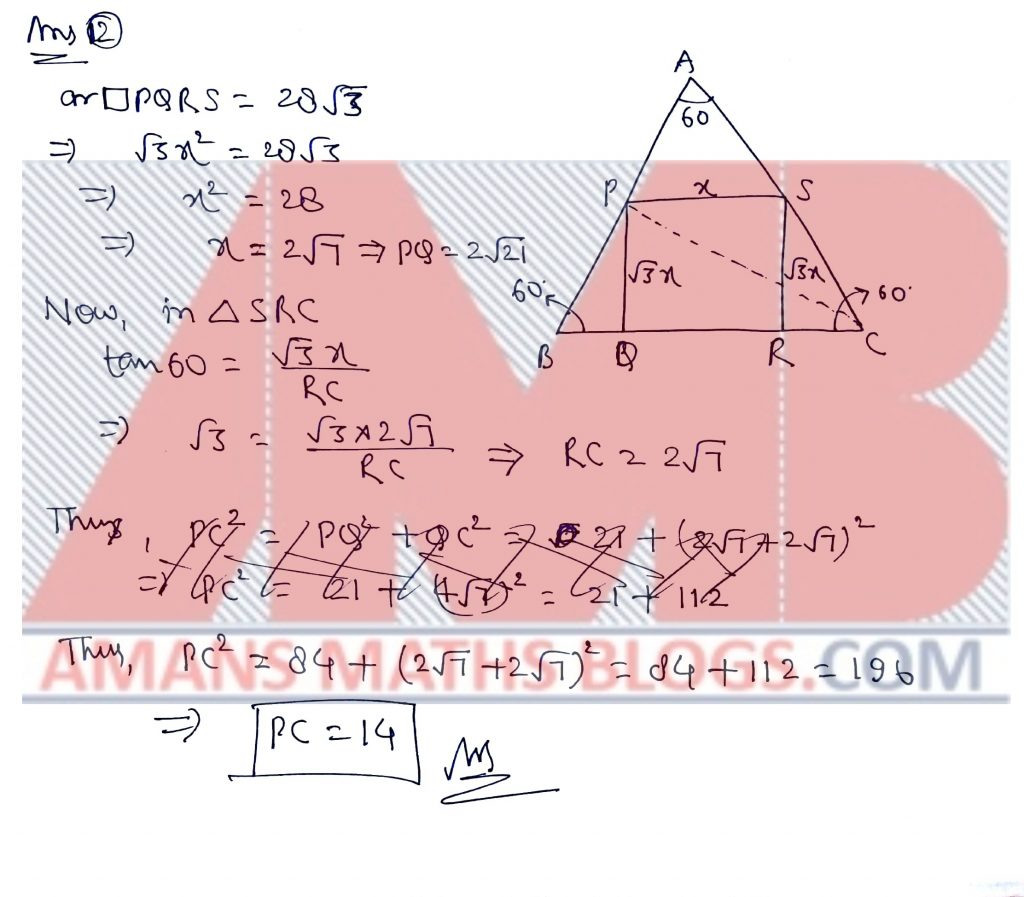Pre RMO Previous Year 2013 Question Paper Question No 13:

To each element of the set S = {1, 2, . . . 1000} a colour is assigned. Suppose that for any two elements a, b of S, if 15 divides a + b then they are both assigned the same colour. What is the maximum possible number of distinct colours used?

Pre RMO Previous Year 2013 Question Paper Solution of Question No 13: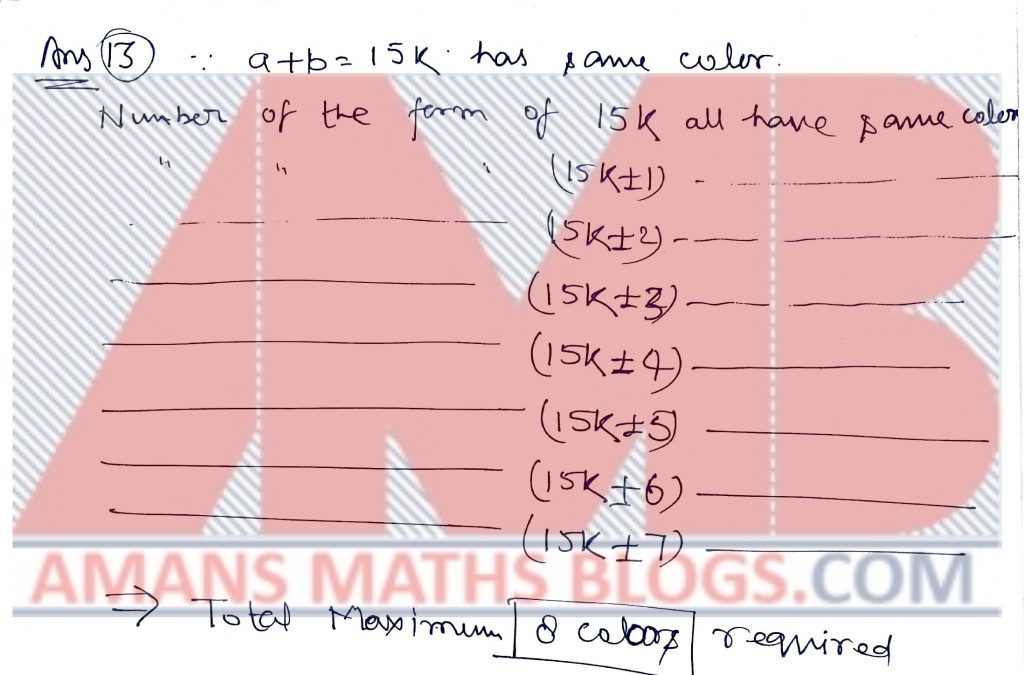Pre RMO Previous Year 2013 Question Paper Question No 14:

Let m be the smallest odd positive integer for which 1 + 2 + . . . + m is a square of an integer and let n be the smallest even positive integer for which 1 + 2 + . . . + n is a square of an integer. What is the value of m + n?

Pre RMO Previous Year 2013 Question Paper Solution of Question No 14: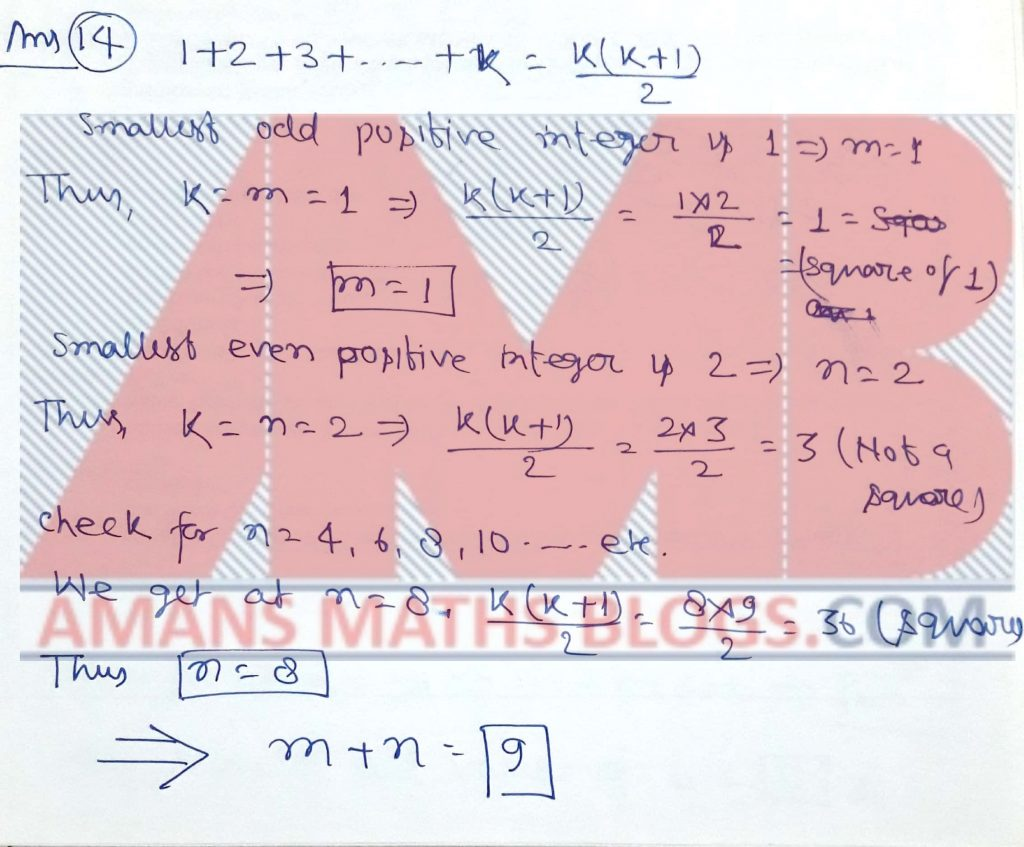Pre RMO Previous Year 2013 Question Paper Question No 15:

Let A1, B1, C1, D1 be the midpoints of the sides of a convex quadrilateral ABCD and let A2, B2, C2, D2 be the midpoints of the sides of the quadrilateral A1B1C1D1. If A2B2C2D2 is a rectangle with sides 4 and 6, then what is the product of the lengths of the diagonals of ABCD?

Pre RMO Previous Year 2013 Question Paper Solution of Question No 15:Pre RMO Previous Year 2013 Question Paper Question No 16:

Let f(x) = x3 – 3x + b and g(x) = x2 + bx – 3, where is a real number. What is the sum of all possible values of b for which the equations f(x) = 0 and g(x) = 0 have a common root?

Pre RMO Previous Year 2013 Question Paper Solution of Question No 16:Pre RMO Previous Year 2013 Question Paper Question No 17:

Let S be a circle with centre O. A chord AB, not a diameter, divides S into two regions R1 and R2 such that O belongs to R2. Let S1 be a circle with centre in R1, touching AB at X and S internally. Let S2 be a circle with centre in R2, touching AB at Y, the circle S internally and passing through the centre of S. The point X lies on the diameter passing through the centre of S2 and angle YXO = 30 degree. If the radius of S2 is 100 then what is the radius of S1?

Pre RMO Previous Year 2013 Question Paper Solution of Question No 17:

Pre RMO Previous Year 2013 Question Paper Question No 18:

What is the maximum possible values of k for which 2013 can be written as a sum of k con-secutive positive integers?

Pre RMO Previous Year 2013 Question Paper Solution of Question No 18:Pre RMO Previous Year 2013 Question Paper Question No 19:

In a triangle ABC with angle BCA = 90*, the perpendicular bisector of AB intersects segments AB and AC at X and Y, respectively. If the ratio of the area of Quadrilateral BXYC to the area of triangle ABC is 13 : 18 and BC = 12 then what is the length of AC?

Pre RMO Previous Year 2013 Question Paper Solution of Question No 19: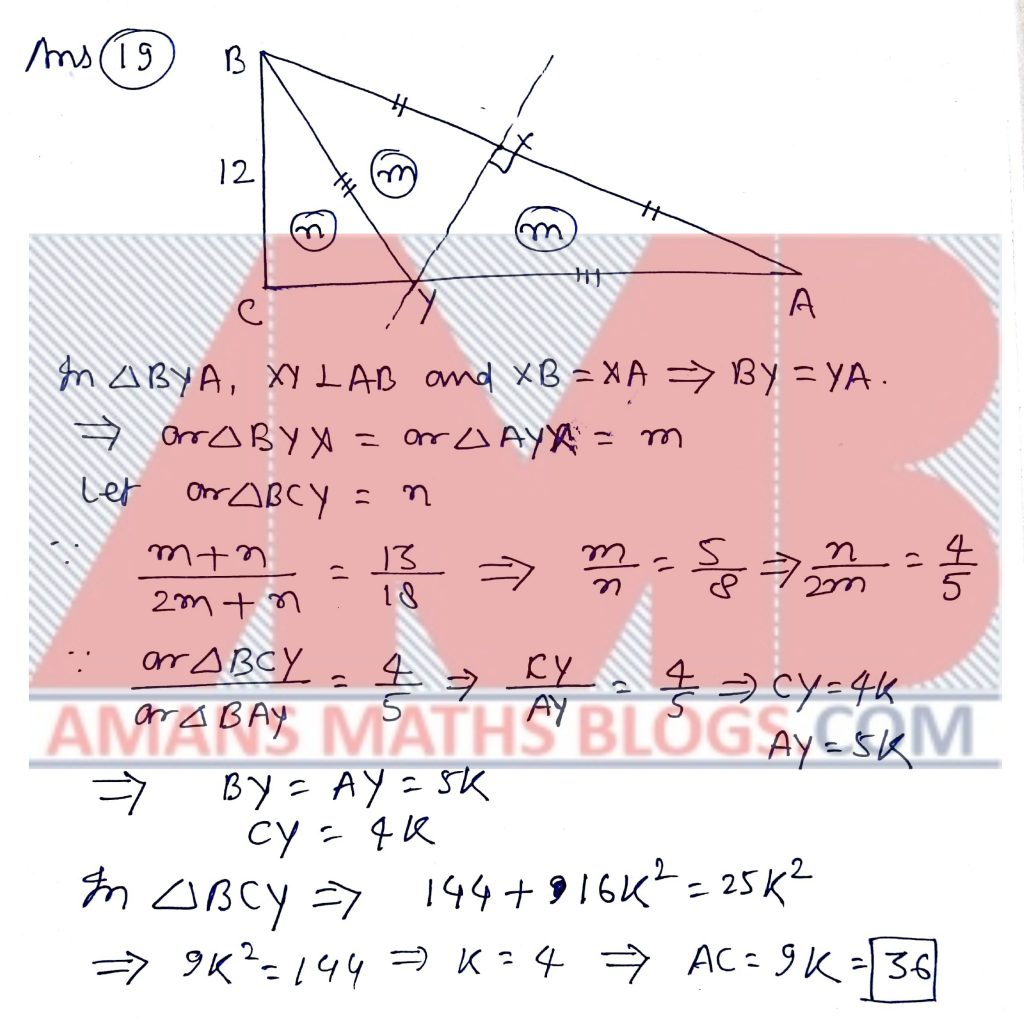Pre RMO Previous Year 2013 Question Paper Question No 20:

What is the sum (in base 10) of all the natural numbers less than 64 which have exactly three ones in their base 2 representation?

Pre RMO Previous Year 2013 Question Paper Solution of Question No 20:

Get Combined Pre RMO 2012 to 2018 Questions Paper with Solution (Click on Image)
error: Content is protected !!